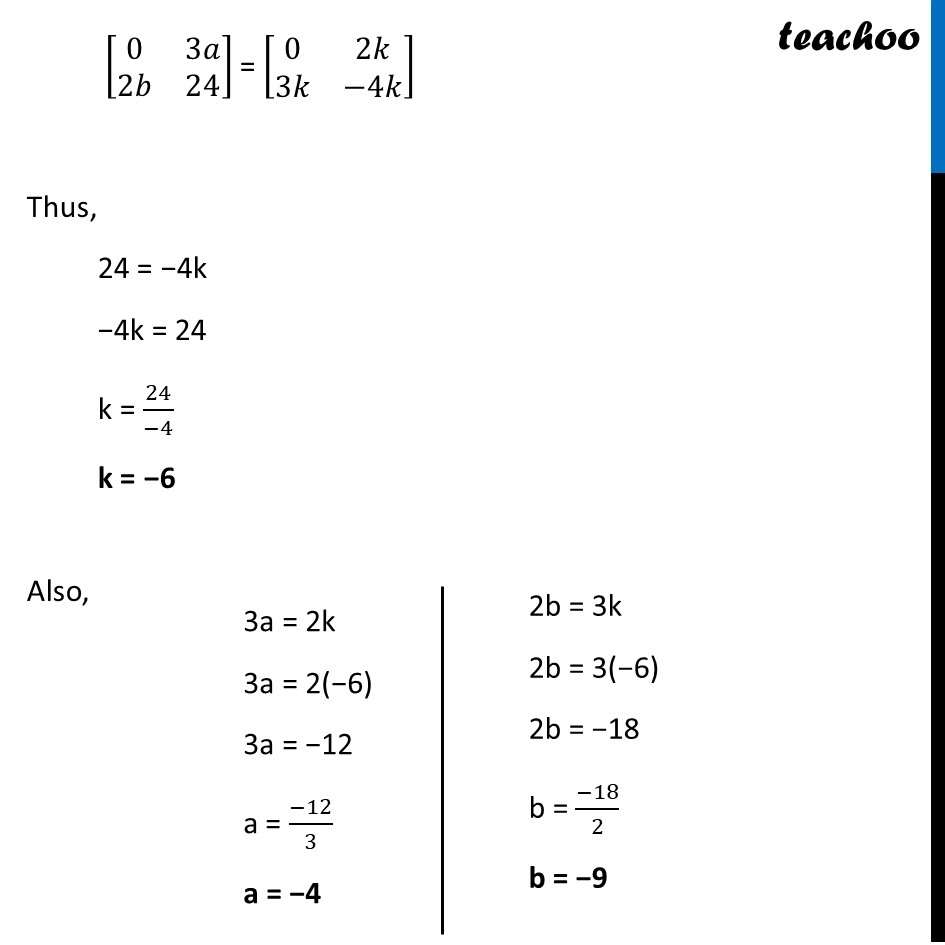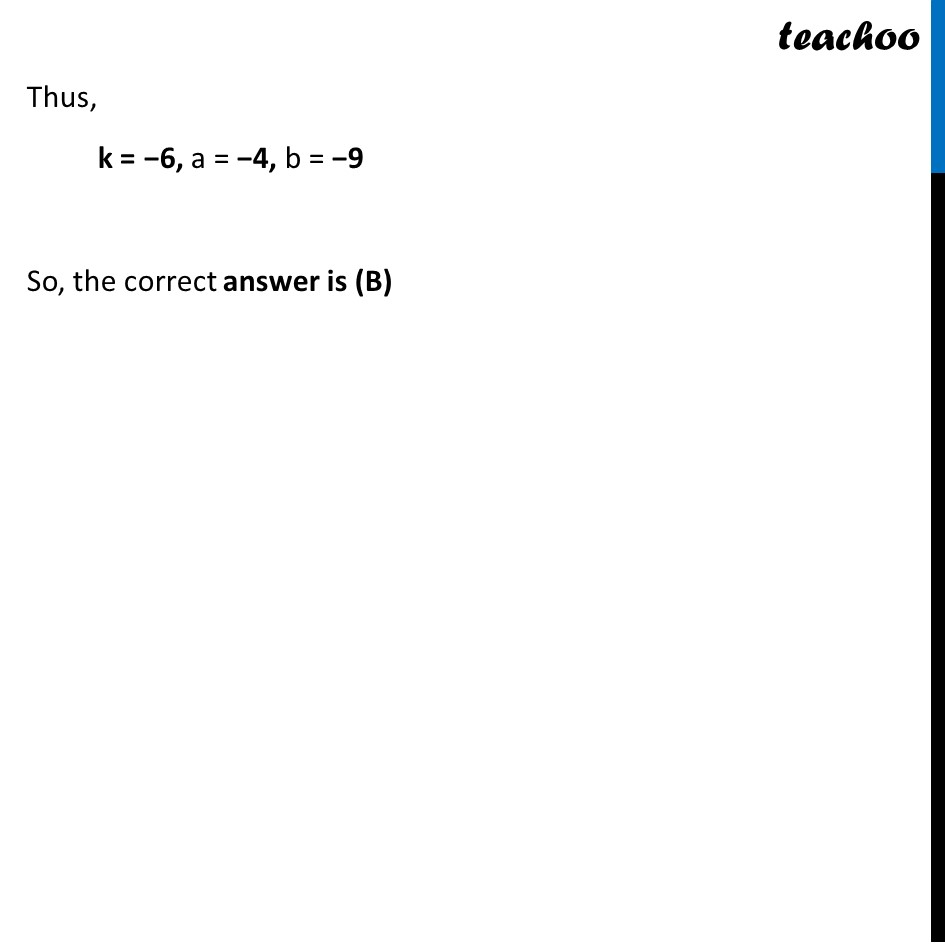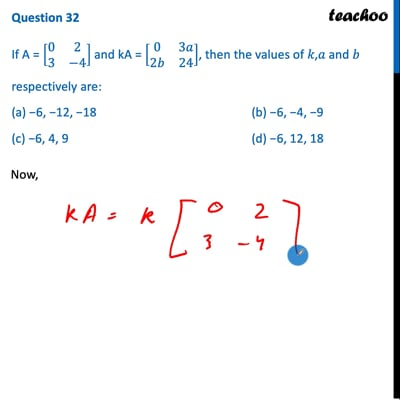CBSE Class 12 Sample Paper for 2022 Boards (MCQ Based - for Term 1)

Class 12
Solutions of Sample Papers and Past Year Papers - for Class 12 Boards

## (c) −6, 4, 9                   (d) −6, 12, 18This video is only available for Teachoo black users

Learn in your speed, with individual attention - Teachoo Maths 1-on-1 Class

### Transcript

Question 32 If A = [■8(0&2@3&−4)] and kA = [■8(0&3𝑎@2𝑏&24)], then the values of 𝑘,𝑎 and 𝑏 respectively are: (a) −6, −12, −18 (b) −6, −4, −9 (c) −6, 4, 9 (d) −6, 12, 18 Now, A = [■8(0&2@3&−4)] kA = k [■8(0&2@3&−4)] kA = [■8(0 × 𝑘&2 × 𝑘@3 × 𝑘&−4 × 𝑘)] kA = [■8(0&2𝑘@3𝑘&−4𝑘)] Putting value of kA [■8(0&3𝑎@2𝑏&24)] = [■8(0&2𝑘@3𝑘&−4𝑘)] Thus, 24 = −4k −4k = 24 k = 24/(−4) k = −6 Also, 3a = 2k 3a = 2(−6) 3a = −12 a = (−12)/3 a = −4 So, the correct answer is (B) [■8(0&3𝑎@2𝑏&24)] = [■8(0&2𝑘@3𝑘&−4𝑘)] Thus, 24 = −4k −4k = 24 k = 24/(−4) k = −6 Also, 3a = 2k 3a = 2(−6) 3a = −12 a = (−12)/3 a = −4 2b = 3k 2b = 3(−6) 2b = −18 b = (−18)/2 b = −9 Thus, k = −6, a = −4, b = −9 So, the correct answer is (B)